# C语言指针-从底层原理到花式技巧，用图文和代码帮你讲解透彻1. 内存地址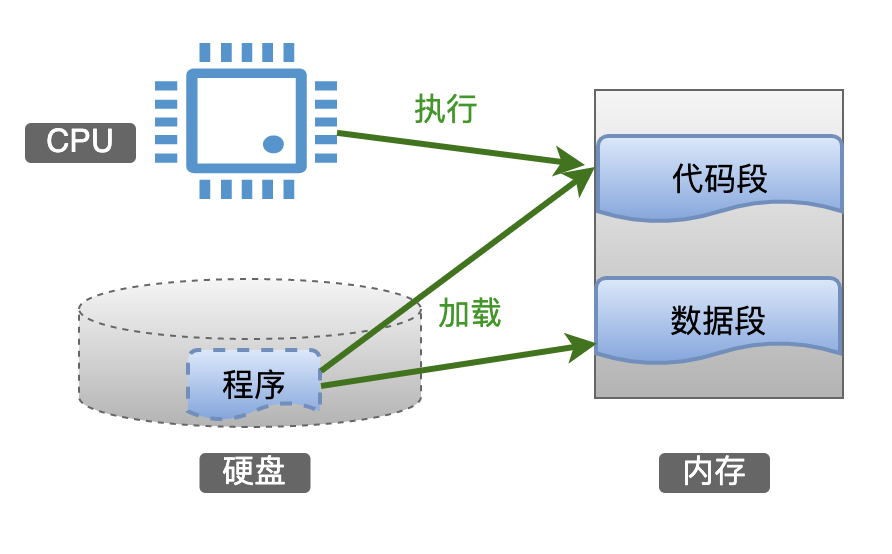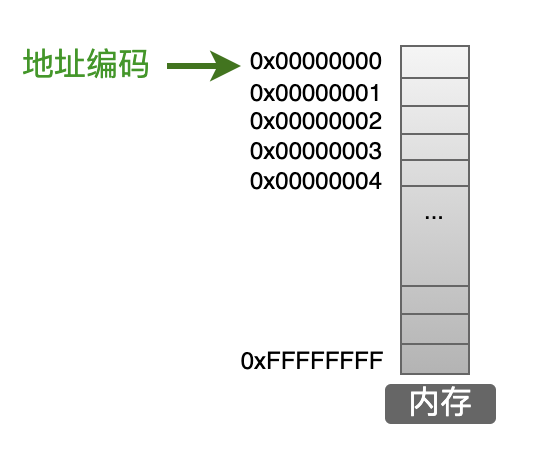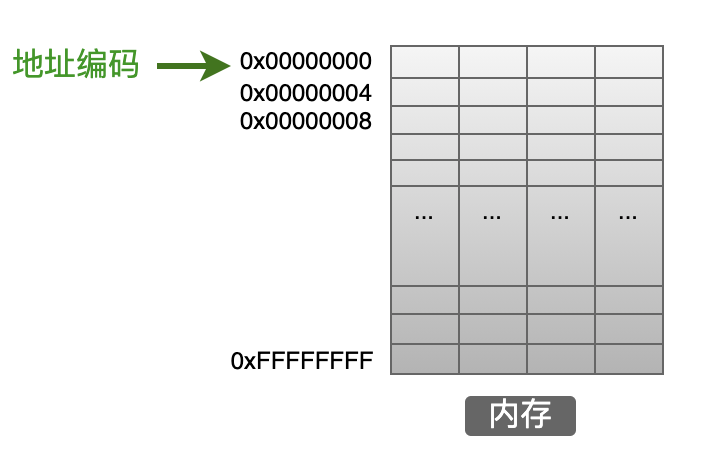2. 32位与64位系统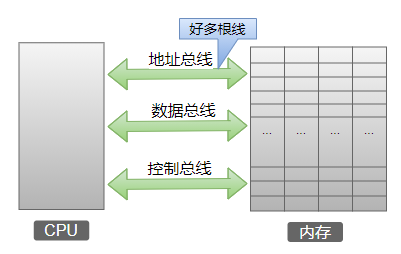【注意】：这里只是描述地址总线的概念，实际的计算机中地址计算方式要复杂的多，比如：虚拟内存中采用分段、分页、偏移量来定位实际的物理内存，在分页中还有大页、小页之分，感兴趣的同学可以自己查一下相关资料。

3. 变量

int a = 20;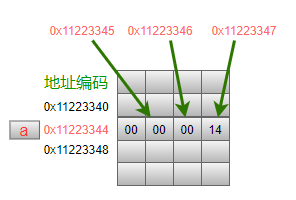char: 1个字节；
short int: 2个字节；
int: 4个字节；
long: 4个字节。

char: 1个字节；
short int: 2个字节；
int: 4个字节；
long: 8个字节。

printf("&a = 0x%x ", &a);

4. 指针变量

int a = 20;
int *pa;
pa = &a;
printf("value = %d ", *pa);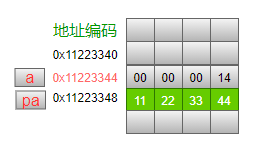&：取地址操作符，用来获取一个变量的地址。上面代码中&a就是用来获取变量a在内存中的存储地址，也就是0x11223344。
*：这个操作符用在2个场景中：定义一个指针的时候，获取一个指针所指向的变量值的时候。

int pa; 这个语句中的表示定义的变量pa是一个指针，前面的int表示pa这个指针指向的是一个int类型的变量。不过此时我们没有给pa进行赋值，也就是说此刻pa对应的存储单元中的4个字节里的值是没有初始化的，可能是0x00000000，也可能是其他任意的数字，不确定；
printf语句中的*表示获取pa指向的那个int类型变量的值，学名叫解引用，我们只要记住是获取指向的变量的值就可以了。
5. 操作指针变量

5.1 指针变量自身的值

int a = 20;这个语句是定义变量a，在随后的代码中，只要写下a就表示要操作变量a中存储的值，操作有两种：读和写。

printf("a = %d ", a); 这个语句就是要读取变量a中的值，当然是20；
a = 100;这个语句就是要把一个数值100写入到变量a中。

printf("pa = %d ", pa); 这个语句就是要读取指针变量pa中的值，当然是0x11223344；
pa = &a;这个语句就是要把新的值写入到指针变量pa中。再次强调一下，指针变量中存储的是地址，如果我们可以提前知道变量a的地址是 0x11223344，那么我们也可以这样来赋值:pa = 0x11223344;

5.2 获取指针变量所指向的数据

5.3 以什么样的数据类型来使用/解释指针变量所指向的内容

int a = 30000;
int *pa = &a;
printf("value = %d ", *pa);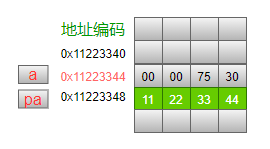char *pc = 0x11223344;
printf("value = %d ", *pc);

1. const属性

const标识符用来表示一个对象的不可变的性质，例如定义：

const int b = 20;

int a = 20;
int b = 20;
int * const p = &a;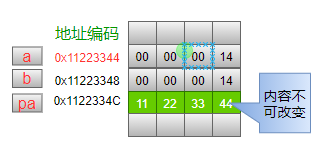int a = 20;
int b = 20;
const int *p = &a;
p = &b;

short int getDataCRC(const char *pData, int len)
{
short int crc = 0x0000;
// 计算CRC
return crc;
}
2. void型指针

int a = 20;
int b = a;
int *p1 = &a;
int *p2 = p1;

int a = 20;
int *p1 = &a;
char *p2 = (char *)p1;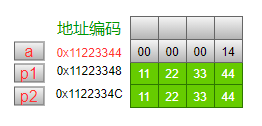p1指针指向的是int型数据，现在想把它的值(0x11223344)赋值给p2，但是由于在定义p2指针时规定它指向的数据类型是char型，因此需要把指针p1进行强制类型转换，也就是把地址0x11223344处的数据按照char型数据来看待，然后才可以赋值给p2指针。

int a = 20;
int *p1 = &a;
void *p2 = p1;

int a = 20;
int *p1 = &a;
void *p2 = p1;
int *p3 = (int *)p2;

void* memcpy(void* dest, const void* src, size_t len);

3. 空指针和野指针

3.1 空指针：不指向任何东西的指针

#ifdef __cplusplus
#define NULL 0
#else
#define NULL ((void *)0)
#endif
3.2 野指针：地址已经失效的指针

int *p = (int *)malloc(4);
*p = 20;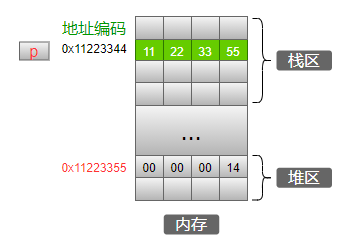int *p = (int *)malloc(4);
*p = 20;
free(p);
// 在free之后就不可以再操作p指针中的数据了。
p = NULL; // 最好加上这一句。

1. 数值型指针

2. 字符串指针

name1在内存中占据8个字节，其中存储了8个字符的ASCII码值；name2在内存中占据9个字节，因为除了存储8个字符的ASCII码值，在最后一个字符"n"的后面还额外存储了一个""，用来标识字符串结束。

char *name2 = "zhangsan";
char *p = name2;
while (*p != "")
{
printf("%c ", *p);
p = p + 1;
}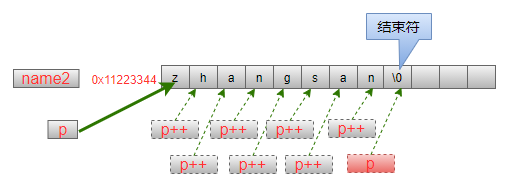printf("%c ", *p++);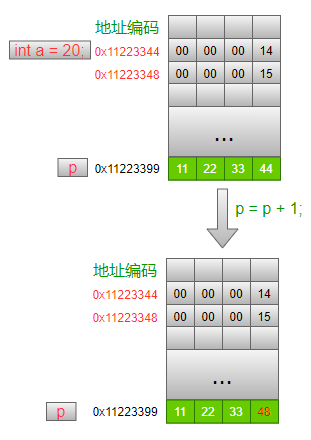int a = {1, 2, 3};
void *p = a;
printf("1: p = 0x%x ", p);
p = p + 1;
printf("2: p = 0x%x ", p);

1: p = 0x733748c0
2: p = 0x733748c1

3. 指针数组与数组指针

int *p1; // 指针数组
int (*p2); // 数组指针
3.1 指针数组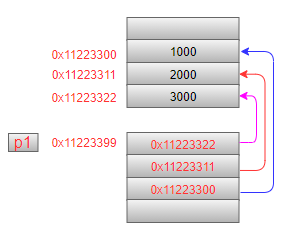int a = 1, b = 2, c = 3;
char *p1;
p1 = &a;
p1 = &b;
p1 = &c;
3.2 数组指针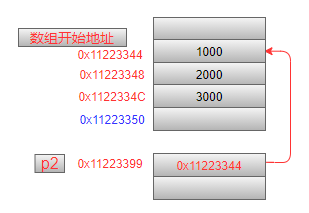int a = {1, 2, 3};
int (*p2) = a;
printf("0x%x ", a);
printf("0x%x ", &a);
printf("0x%x ", p2);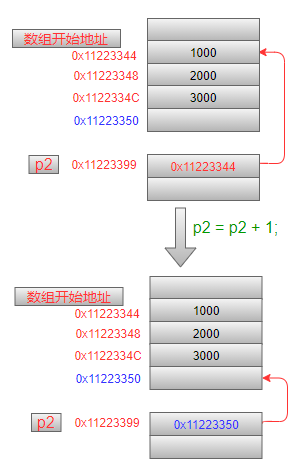4. 二维数组和指针

int a = {{1,2,3}, {4,5,6}, {7,8,9}}; // 二维数组
int (*p0) = NULL; // p0是一个指针，指向一个数组
int (*p1) = NULL; // p1是一个指针，指向一个数组
int (*p2) = NULL; // p2是一个指针，指向一个数组
p0 = a;
p1 = a;
p2 = a;
printf("0: %d %d %d ", *(*p0 + 0), *(*p0 + 1), *(*p0 + 2));
printf("1: %d %d %d ", *(*p1 + 0), *(*p1 + 1), *(*p1 + 2));
printf("2: %d %d %d ", *(*p2 + 0), *(*p2 + 1), *(*p2 + 2));

0: 1 2 3
1: 4 5 6
2: 7 8 9

5. 结构体指针

C语言中的基本数据类型是预定义的，结构体是用户定义的，在指针的使用上可以进行类比，唯一有区别的就是在结构体指针中，需要使用->箭头操作符来获取结构体中的成员变量，例如：

typedef struct
{
int age;
char name;
} Student;
Student s;
s.age = 20;
strcpy(s.name, "lisi");
Student *p = &s;
printf("age = %d, name = %s ", p->age, p->name);

Student s;
Student *p = &s;
printf("size of Student = %d ", sizeof(Student));
printf("1: 0x%x, 0x%x ", s, p);
p++;
printf("2: 0x%x ", p);

size of Student = 12
1: 0x4c02ac00, 0x4c02ac00
2: 0x4c02ac0c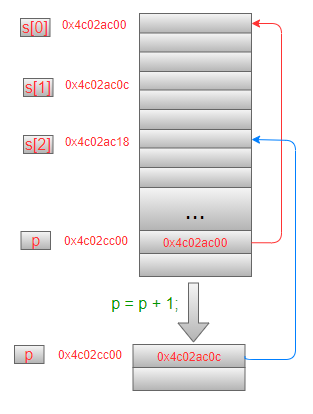6. 函数指针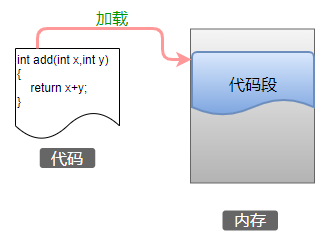int add(int x,int y)
{
return x+y;
}
int main()
{
int a = 1, b = 2;
int (*p)(int, int);
p = add;
printf("%d + %d = %d ", a, b, p(a, b));
}

int (*p)(int, int);这条语句就是用来定义一个函数指针，它指向一个函数，这个函数必须符合下面这2点(学名叫：函数签名)：

int (*p)(int, int);
int (*p2)(int, int);

typedef int (*pFunc)(int, int);

int add(int a, int b) { return a + b; }
int sub(int a, int b) { return a - b; }
int mul(int a, int b) { return a * b; }
int divide(int a, int b) { return a / b; }
int main()
{
int a = 4, b = 2;
int (*p)(int, int);
p = add;
p = sub;
p = mul;
p = divide;
printf("%d + %d = %d ", a, b, p(a, b));
printf("%d - %d = %d ", a, b, p(a, b));
printf("%d * %d = %d ", a, b, p(a, b));
printf("%d / %d = %d ", a, b, p(a, b));
}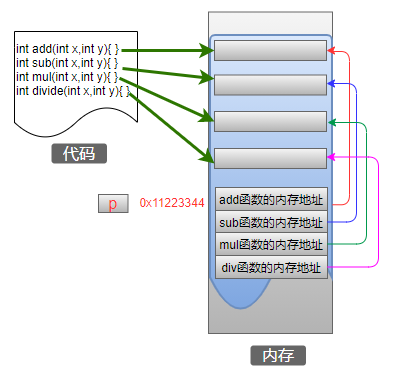【原创声明】

B站：道哥分享

CSDN：道哥分享原来gdb的底层调试原理这么简单
 生产者和消费者模式中的双缓冲技术
 深入LUA脚本语言，让你彻底明白调试原理
 一步步分析-如何用C实现面向对象编程
 关于加密、证书的那些事

## Docker RabbitMQ日志映射

RabbitMQDocker日志映射的坑最近在做公司项目使用docker安装rabbitMQ的时候需要映射日志路径出来，查阅文档文档可以直接配置环境变量RABBITMQ_LOG_BASE...

## LeetCode242.有效的字母异位词

LeetCode242.有效的字母异位词1.题目描述：给定两个字符串s和t，编写一个函数来判断t是否是s的字母异位词。示例1:输入:s=“anagram”,t...

...

## SOX源代码分析

0.流程input->balance->effectsbalance?每次都会有?目的?draineffectfloweffect一共有5个effect floweffect后out_fl...